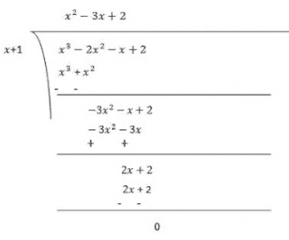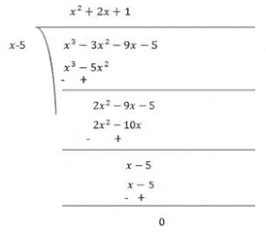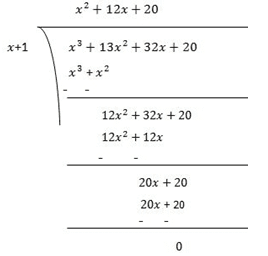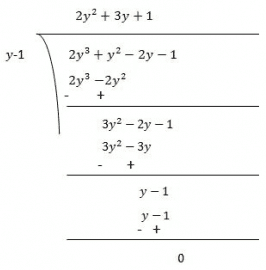NCERT Solutions - Polynomials (Exercise 2.3)

# Polynomials (Exercise 2.3) NCERT Solutions - Mathematics (Maths) Class 10

Q1. Determine which of the following polynomials has (x + 1) a factor:
(i) x+ x+ x + 1

Ans: Let p(x) = x+ x+ x + 1
The zero of x + 1 is -1. [x + 1 = 0 means x = -1]
p(−1) = (−1)+ (−1)+ (−1) + 1
= −1 + 1 − 1 + 1
= 0
∴ By factor theorem, x + 1 is a factor of x+ x+ x + 1

(ii) x+ x+ x+ x + 1
Ans: Let p(x) =  x+ x+ x+ x + 1
The zero of x + 1 is -1. . [x + 1= 0 means x = -1]
p(−1) = (−1)4 + (−1)+ (−1)+ (−1) + 1
= 1 − 1 + 1 − 1 + 1
= 1 ≠ 0
∴ By factor theorem, x+1 is not a factor of x+ x+ x+ x + 1

(iii) x+ 3x+ 3x+ x + 1
Ans: Let p(x)= x+ x+ x+ x + 1
The zero of x+1 is -1.
p(−1)=(−1)4+3(−1)3+3(−1)2+(−1)+1
=1−3+3−1+1
=1 ≠ 0
∴ By factor theorem, x + 1 is not a factor of x4 +3x+ 3x2 + x + 1

(iv) x3 – x2– (2+√2)x +√2
Ans: Let p(x) = x3–x2–(2+√2)x +√2
The zero of x+1 is -1.
p(−1) = (-1)3–(-1)2–(2+√2)(-1) + √2 = −1−1+2+√2+√2
= 2√2 ≠ 0
∴ By factor theorem, x+1 is not a factor of x3–x2–(2+√2)x +√2

Q2. Use the Factor Theorem to determine whether g(x) is a factor of p(x) in each of the following cases:
(i) p(x) = 2x+ x2 – 2x – 1, g(x) = x + 1
Ans: p(x) = 2x3+x2–2x–1, g(x) = x+1
g(x) = 0
⇒ x+1 = 0
⇒ x = −1
∴ Zero of g(x) is -1.
Now,
p(−1) = 2(−1)3+(−1)2–2(−1)–1
= −2 + 1 + 2 − 1
= 0
∴ By factor theorem, g(x) is a factor of p(x).

(ii) p(x) = x+ 3x+ 3x + 1, g(x) = x + 2
Ans: p(x) = x3+ 3x3x + 1, g(x) = x + 2
g(x) = 0
⇒ x + 2 = 0
⇒ x = −2
∴ Zero of g(x) is -2.
Now,
p(−2) = (−2)3+3(−2)2+3(−2)+1
= −8 + 12 − 6 + 1
= −1 ≠ 0
∴ By factor theorem, g(x) is not a factor of p(x).

(iii) p(x)= x– 4x+ x + 6, g(x) = x – 3
Ans: p(x) = x3– 4x+ x + 6, g(x) = x - 3
g(x) = 0
⇒ x−3 = 0
⇒ x = 3
∴ Zero of g(x) is 3.
Now,
p(3) = (3)3−4(3)+ (3) + 6
= 27 − 36 + 3 + 6
= 0
∴ By factor theorem, g(x) is a factor of p(x).

Q3. Find the value of k, if x–1 is a factor of p(x) in each of the following cases:
(i) p(x) = x+ x + k
Ans: If x - 1 is a factor of p(x), then p(1) = 0
By Factor Theorem
⇒ (1)2+(1)+k = 0
⇒ 1+1+k = 0
⇒ 2+k = 0
⇒ k = −2

(ii) p(x) = 2x+ kx + √2
Ans:
If x-1 is a factor of p(x), then p(1)=0
⇒ 2(1)+ k(1) + √2 = 0
⇒ 2 + k + √2 = 0
⇒ k = −(2 + √2)

(iii) p(x) = kx2–√2x + 1
Ans: If x - 1 is a factor of p(x), then p(1)=0
By Factor Theorem
⇒ k(1)2-√2(1)+1=0
⇒ k = √2-1

(iv) p(x) = kx– 3x + k
Ans: If x-1 is a factor of p(x), then p(1) = 0
By Factor Theorem
⇒ k(1)2–3(1)+k = 0
⇒ k−3+k = 0
⇒ 2k−3 = 0
⇒ k= 3/2

Q4. Factorize:
(i) 12x– 7x + 1
Ans: Using the splitting the middle term method,
We have to find a number whose sum = -7 and product =1×12 = 12
We get -3 and -4 as the numbers [-3+-4=-7 and -3×-4 = 12]
12x2–7x+1= 12x2-4x-3x+1
= 4x(3x-1)-1(3x-1)
= (4x-1)(3x-1)

(ii) 2x+ 7x + 3
Ans: Using the splitting the middle term method,
We have to find a number whose sum = 7 and product = 2×3 = 6
We get 6 and 1 as the numbers [6+1 = 7 and 6×1 = 6]
2x2+7x+3 = 2x2+6x+1x+3
= 2x (x+3)+1(x+3)
= (2x+1)(x+3)

(iii) 6x+ 5x - 6
Ans: Using the splitting the middle term method,
We have to find a number whose sum = 5 and product = 6×-6 = -36
We get -4 and 9 as the numbers [-4+9 = 5 and -4×9 = -36]
6x2+5x-6 = 6x2+9x–4x–6
= 3x(2x+3)–2(2x+3)
= (2x+3)(3x–2)

(iv) 3x2–x–4
Ans: Using the splitting the middle term method,
We have to find a number whose sum = -1 and product = 3 × -4 = -12
We get -4 and 3 as the numbers [-4 + 3 = -1 and -4 × 3 = -12]
3x– x – 4 = 3x– x – 4
= 3x– 4x + 3x – 4
= x(3x – 4) + 1(3x – 4)
= (3x – 4)(x + 1)

5. Factorize:
(i) x3– 2x– x + 2
Ans: Let p(x) = x3–2x2–x+2
Factors of 2 are ±1 and ± 2
Now,
p(x) = x3–2x2–x+2
p(−1) = (−1)3–2(−1)2–(−1)+2
= −1−2+1+2
= 0
Therefore, (x+1) is the factor of p(x)Now, Dividend = Divisor × Quotient + Remainder
Now by splitting the middle term method,
(x+1)(x2–3x+2) = (x+1)(x2–x–2x+2)
= (x+1)(x(x−1)−2(x−1))
= (x+1)(x−1)(x-2)

(ii) x– 3x– 9x – 5
Ans: Let p(x) = x3–3x2–9x–5
Factors of 5 are ±1 and ±5
By trial method, we find that
p(5) = 0
So, (x-5) is factor of p(x)
Now,
p(x) = x3–3x2–9x–5
p(5) = (5)3–3(5)2–9(5)–5
= 125−75−45−5
= 0
Therefore, (x-5) is the factor of  p(x)Now, Dividend = Divisor × Quotient + Remainder
(x−5)(x2+2x+1) = (x−5)(x2+x+x+1)
= (x−5)(x(x+1)+1(x+1))
= (x−5)(x+1)(x+1)

(iii) x+ 13x+ 32x + 20
Ans: Let p(x) = x3+13x2+32x+20
Factors of 20 are ±1, ±2, ±4, ±5, ±10 and ±20
By trial method, we find that
p(-1) = 0
So, (x+1) is factor of p(x)
Now,
p(x)= x3+13x2+32x+20
p(-1) = (−1)3+13(−1)2+32(−1)+20
= −1+13−32+20
= 0
Therefore, (x+1) is the factor of p(x)Now, Dividend = Divisor × Quotient +Remainder
Now by splitting the middle term method,
(x+1)(x2+12x+20) = (x+1)(x2+2x+10x+20)
= (x−5)x(x+2)+10(x+2)
= (x−5)(x+2)(x+10)

(iv) 2y+ y– 2y – 1
Ans: Let p(y) = 2y3+y2–2y–1
Factors = 2×(−1)= -2 are ±1 and ±2
By trial method, we find that
p(1) = 0
So, (y-1) is factor of p(y)
Now,
p(y) = 2y3+y2–2y–1
p(1) = 2(1)3+(1)2–2(1)–1
= 2+1−2
= 0
Therefore, (y-1) is the factor of p(y)Now, Dividend = Divisor × Quotient + Remainder
Now by splitting the middle term method,
(y−1)(2y2+3y+1) = (y−1)(2y2+2y+y+1)
= (y−1)(2y(y+1)+1(y+1))
= (y−1)(2y+1)(y+1)

The document Polynomials (Exercise 2.3) NCERT Solutions | Mathematics (Maths) Class 10 is a part of the Class 10 Course Mathematics (Maths) Class 10.
All you need of Class 10 at this link: Class 10

## Mathematics (Maths) Class 10

115 videos|478 docs|129 tests

## FAQs on Polynomials (Exercise 2.3) NCERT Solutions - Mathematics (Maths) Class 10

 1. What are polynomials?Ans. Polynomials are mathematical expressions consisting of variables, coefficients, and exponents, combined using arithmetic operations such as addition, subtraction, multiplication, and division. In simpler terms, polynomials are algebraic expressions that involve one or more variables and have non-negative integer exponents.
 2. What is the degree of a polynomial?Ans. The degree of a polynomial is the highest power of the variable present in the polynomial. For example, the degree of the polynomial 3x^2 + 5x + 1 is 2, as the highest power of x in the polynomial is 2. The degree of a polynomial helps in determining its nature and behavior.
 3. How can we add or subtract polynomials?Ans. To add or subtract polynomials, we need to combine the like terms. Like terms are those terms that have the same variable and the same power. For example, in the polynomial 3x^2 + 5x + 1 + 2x^2 + 4x - 3, the like terms are 3x^2 and 2x^2, 5x and 4x, and 1 and -3. By combining the like terms, we get 5x^2 + 9x - 2.
 4. What is synthetic division?Ans. Synthetic division is a method used to divide a polynomial by a linear polynomial of the form (x - a). It is a shortcut method that involves writing only the coefficients of the polynomial and performing a series of arithmetic operations. Synthetic division is useful in finding the quotient and remainder of a polynomial division, and is often used in solving polynomial equations and factorization.
 5. How do we find the zeroes of a polynomial?Ans. The zeroes of a polynomial are the values of the variable that make the polynomial equal to zero. To find the zeroes of a polynomial, we need to solve the equation P(x) = 0, where P(x) is the polynomial. There are various methods to find the zeroes of a polynomial, such as factorization, synthetic division, and using the quadratic formula. The zeroes of a polynomial are also known as roots or solutions of the polynomial equation.

## Mathematics (Maths) Class 10

115 videos|478 docs|129 testsExplore Courses for Class 10 examSignup to see your scores go up within 7 days! Learn & Practice with 1000+ FREE Notes, Videos & Tests.
10M+ students study on EduRev
Track your progress, build streaks, highlight & save important lessons and more!
Related Searches

,

,

,

,

,

,

,

,

,

,

,

,

,

,

,

,

,

,

,

,

,

;